Perimeter of Rectangle

Chapter 10 Class 6 Mensuration
Concept wise

Let’s take a rectangle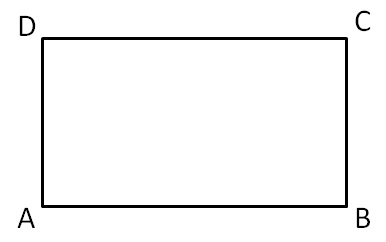We know that

In a rectangle, opposite sides are equal

AB = CD

Let

AB = Length of rectangle = l

BC = Breadth of rectangle = b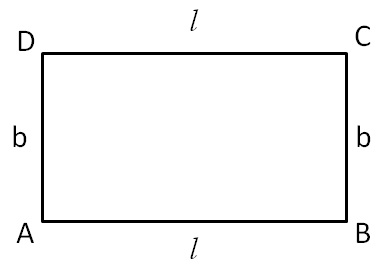So,

AB = CD = l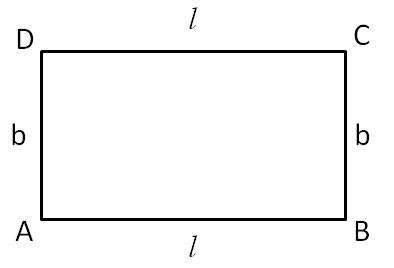Now,

Perimeter of rectangle = Sum of all sides

= AB + BC + CD + DA

= l + b + l + b

= 2 l + 2b

= 2 ( l + b)

So,

Perimeter of rectangle = 2 ( l + b)

Let’s take some examples

#### Find perimeter of rectangle where length is 15cm & breadth is 9 cm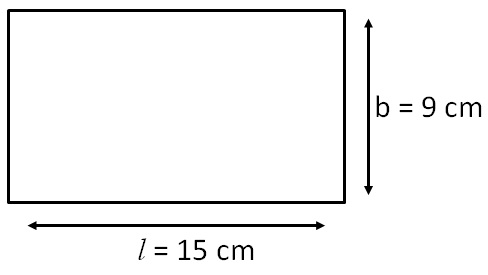Given,

Length = l = 15 cm

Breadth = b = 9 cm

Perimeter of rectangle = 2 × (Length + Breadth)

= 2 × (15 + 9)

= 2 × 24

= 48 cm

So, perimeter of rectangle is 48 cm

#### Find perimeter of rectangle where length & breadth are 0.5 m & 0.2 m respectively.

Given,

Length = l = 0.5 cm

Breadth = b = 0.2 cm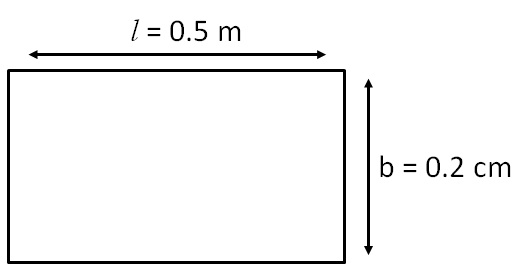Perimeter of rectangle = 2 × (Length + Breadth)

= 2 × (0.5 + 0.2)

= (2 × 0.7)

= 1.4 m

= 1.4 × 100 cm               (1m = 100 cm)

= 140 cm

Learn in your speed, with individual attention - Teachoo Maths 1-on-1 Class